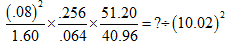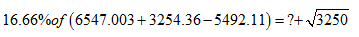New Students Offer - Use Code HELLO

# Approximation Questions With Solutions Part-1

1. What should come at the place of question mark (?) in the following question?
A. 145
B. 160
C. 155
D. 130
E. 170

2. What should come at the place of question mark (?) in the following question?A. 8
B. 5
C. 3
D. 1
E. 11

3. What should come at the place of question mark (?) in the following question?A. 5
B. 15
C. 25
D. 12
E. 23

4. What should come at the place of question mark (?) in the following question?A. 4
B. 5
C. 6
D. 3
E. 2

5. What should come at the place of question mark (?) in the following question?A. 620
B. 690
C. 660
D. 720
E. 750

1. B

2. C

3. A

4. E

5. C